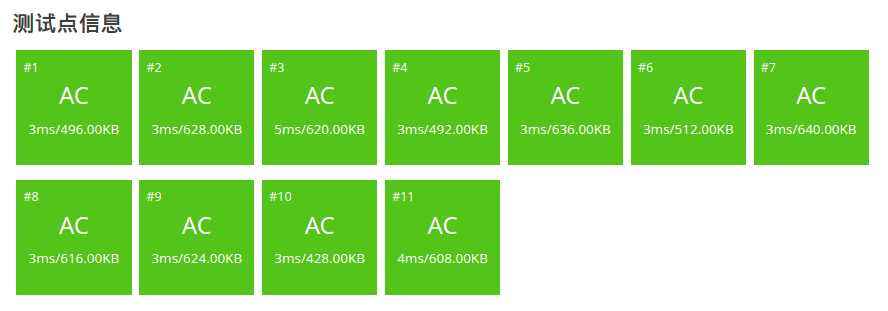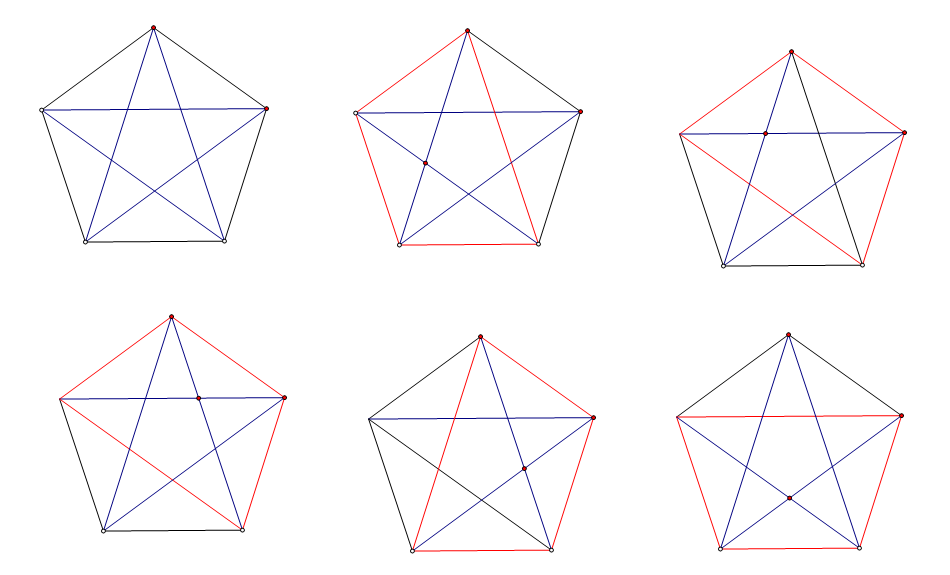# 【入门1】顺序结构 P2181 对角线 数学结论+过程溢出,结果不溢出的处理

【入门1】顺序结构 P2181 对角线

【入门1】顺序结构n=10^5,C(10^5,4)=10^5*(10^5-1)*(10^5-2)*(10^5-3)/(4*3*2*1)=4*10^18

2^63-1=9.0*10^18

AC代码如下：

#include <bits/stdc++.h>
#define LL long long
LL a;
int main(){
LL n,ans=1;
int i;
scanf("%lld",&n);
for(i=0;i<4;i++)a[i]=n-i;
for(i=0;i<4;i++)
if(a[i]%3==0){a[i]/=3;break;}
for(i=0;i<4;i++)
if(a[i]%4==0){a[i]/=4;break;}
for(i=0;i<4;i++)
if(a[i]%2==0){a[i]/=2;break;}
for(i=0;i<4;i++)ans*=a[i];
printf("%lld ",ans);
return 0;
}